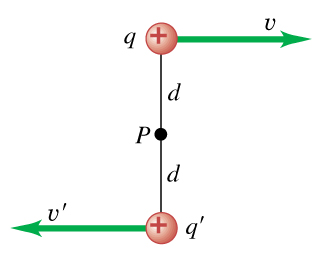# Problem: Positive point charges q = 9.00 μC and q' = μ4.00 C are moving relative to an observer at point P, as shown in the figure . The distance d is 0.130 m ,v = 4.60×106 m/s , and v' = 9.20×106 m/s .A. When the two charges are at the locations shown in the figure, what is the magnitude of the net magnetic field they produce at point P?B. What is the magnitude of the electric force that each charge exerts on the other?C. If the direction of v is reversed, so both charges are moving in the same direction, what is the magnitude of the magnetic forces that the two charges exert on each other?D. What is the direction of the net magnetic field at point P?E. What is the direction of the electric force that each charge exerts on the other?F. What is the magnitude of the magnetic force that each charge exerts on the other?G. What is the direction of the magnetic force that each charge exerts on the other?H. What is the ratio of the magnitude of the electric force to the magnitude of the magnetic force?I. What is the direction of the magnetic forces that the two charges exert on each other?

###### FREE Expert Solution

The magnetic field at a moving point charge is expressed by:

$\overline{){\mathbf{B}}{\mathbf{=}}\frac{{\mathbf{\mu }}_{\mathbf{0}}}{\mathbf{4}\mathbf{\pi }}\frac{\mathbf{q}\mathbf{\left(}\mathbf{v}\mathbf{×}\stackrel{\mathbf{^}}{\mathbf{r}}\mathbf{\right)}}{{\mathbf{r}}^{\mathbf{2}}}}$

A.

The net magnetic field at point P is given by:

B = B1 + B2

We have:

$\begin{array}{rcl}\mathbf{B}& \mathbf{=}& \frac{{\mathbf{\mu }}_{\mathbf{0}}}{\mathbf{4}\mathbf{\pi }}\frac{\mathbf{qv}}{{\mathbf{d}}^{\mathbf{2}}}\mathbf{+}\frac{{\mathbf{\mu }}_{\mathbf{0}}}{\mathbf{4}\mathbf{\pi }}\frac{{\mathbf{q}}^{\mathbf{1}}{\mathbf{v}}^{\mathbf{1}}}{{\mathbf{d}}^{\mathbf{2}}}\\ & \mathbf{=}& \frac{{\mathbf{\mu }}_{\mathbf{0}}}{\mathbf{4}{\mathbf{\pi d}}^{\mathbf{2}}}\mathbf{\left(}{\mathbf{qv}}{\mathbf{+}}{{\mathbf{q}}}^{{\mathbf{1}}}{{\mathbf{v}}}^{{\mathbf{1}}}{\mathbf{\right)}}\\ & \mathbf{=}& \frac{\mathbf{\left(}\mathbf{4}\mathbf{\pi }\mathbf{×}{\mathbf{10}}^{\mathbf{-}\mathbf{7}}\mathbf{\right)}}{\mathbf{\left(}\mathbf{4}\mathbf{\pi }\mathbf{\right)}{\mathbf{\left(}\mathbf{0}\mathbf{.}\mathbf{130}}^{\mathbf{2}}\mathbf{\right)}}\mathbf{\left[}\mathbf{\left(}\mathbf{9}\mathbf{.}\mathbf{00}\mathbf{×}{\mathbf{10}}^{\mathbf{-}\mathbf{6}}\mathbf{\right)}\mathbf{\left(}\mathbf{4}\mathbf{.}\mathbf{6}\mathbf{×}{\mathbf{10}}^{\mathbf{6}}\mathbf{\right)}\mathbf{+}\mathbf{\left(}\mathbf{4}\mathbf{.}\mathbf{00}\mathbf{×}{\mathbf{10}}^{\mathbf{-}\mathbf{6}}\mathbf{\right)}\mathbf{\left(}\mathbf{9}\mathbf{.}\mathbf{2}\mathbf{×}{\mathbf{10}}^{\mathbf{6}}\mathbf{\right)}\mathbf{\right]}\end{array}$###### Problem Details

Positive point charges q = 9.00 μC and q' = μ4.00 C are moving relative to an observer at point P, as shown in the figure. The distance d is 0.130 m ,v = 4.60×106 m/s , and v' = 9.20×106 m/s .

A. When the two charges are at the locations shown in the figure, what is the magnitude of the net magnetic field they produce at point P?

B. What is the magnitude of the electric force that each charge exerts on the other?

C. If the direction of v is reversed, so both charges are moving in the same direction, what is the magnitude of the magnetic forces that the two charges exert on each other?

D. What is the direction of the net magnetic field at point P?

E. What is the direction of the electric force that each charge exerts on the other?

F. What is the magnitude of the magnetic force that each charge exerts on the other?

G. What is the direction of the magnetic force that each charge exerts on the other?

H. What is the ratio of the magnitude of the electric force to the magnitude of the magnetic force?

I. What is the direction of the magnetic forces that the two charges exert on each other?